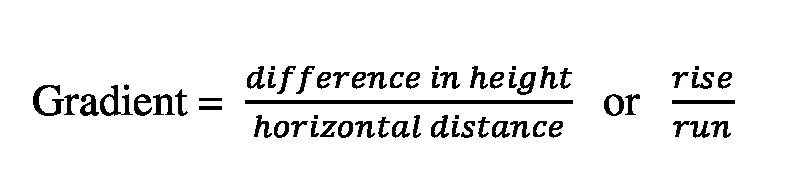Select Page

Gradient is a measure of how steep or gentle a slope is. CXC requires Geography students to be able to calculate the gradient of a slope and express it as a ratio. Fortunately, this is quite simple.

Gradient is calculated using this formula:“Rise over run” may be easier to remember.

Working it out:

Step one: Find the two points you will be working with. Let’s call them point A and point B.

Step two: Find the height of point A and point B by using the contour lines on your map (or trig stations in some cases). Let’s imagine that point A is 50 meters above seal level and point B is 250 meters above sea level. Subtract the smaller number from the larger one to get the difference in height. In this case:

Difference in height = 250 meters – 50 meters = 200 meters

Step 3: Use the scale on your map to measure the horizontal distance from point A to point B. If you do not know how to do this click here. Lets imagine the horizontal distance between points A and B is 3 kilometers. We need the rise and the run to be in the same units before we move on to the next step. We can convert 3 kilometers to meters by multiplying it by 1000 to get 3000 meters.

difference in height/horizontal distance = 200 meters / 3000 meters

Step 5: In order to get a ratio you need to divide both the numerator and the denominator by the difference in height. In our example this works out to be 1/15. We then express this as a ratio. We say the gradient of the slope from point A to point B is 1:15. This is your final answer.

What does this mean?

In our example above, the gradient 1:15 means that on average, as we move from point A to point B, as we go up the slope, we gain one meter of height for every 15 meters we travel forward. If you go down the slope you lose one meter of height for every 15 meters you travel forward. Since this is a ratio, you can freely insert another unit of your choice here such as 1 foot of height for every 15 feet of horizontal distance. Also, bear in mind that the larger the number on the right side of the ratio the gentler the slope is.

Important tip!

You must ensure that the difference in height and the horizontal distance are in the same units before you calculate the ratio. On some maps, heights are given in feet and horizontal distances are given in kilometers or even miles. In order to calculate the gradient you need to know how to convert one unit into another. The information below is useful to know.

One kilometer = 1000 meters

One mile = 5280 feet

One meter = 3.28 feet

One foot = 0.3048 meters

Watch the video below for a demonstration of calculating gradient